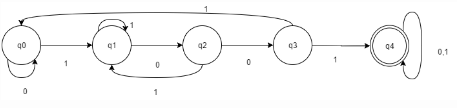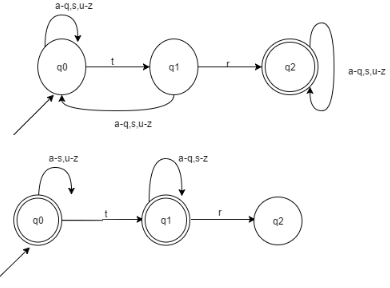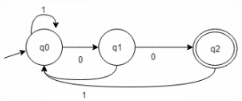Test: Equivalence of NFA & DFA

# Test: Equivalence of NFA & DFA - Computer Science Engineering (CSE)

Test Description

## 10 Questions MCQ Test Theory of Computation - Test: Equivalence of NFA & DFA

Test: Equivalence of NFA & DFA for Computer Science Engineering (CSE) 2023 is part of Theory of Computation preparation. The Test: Equivalence of NFA & DFA questions and answers have been prepared according to the Computer Science Engineering (CSE) exam syllabus.The Test: Equivalence of NFA & DFA MCQs are made for Computer Science Engineering (CSE) 2023 Exam. Find important definitions, questions, notes, meanings, examples, exercises, MCQs and online tests for Test: Equivalence of NFA & DFA below.
Solutions of Test: Equivalence of NFA & DFA questions in English are available as part of our Theory of Computation for Computer Science Engineering (CSE) & Test: Equivalence of NFA & DFA solutions in Hindi for Theory of Computation course. Download more important topics, notes, lectures and mock test series for Computer Science Engineering (CSE) Exam by signing up for free. Attempt Test: Equivalence of NFA & DFA | 10 questions in 10 minutes | Mock test for Computer Science Engineering (CSE) preparation | Free important questions MCQ to study Theory of Computation for Computer Science Engineering (CSE) Exam | Download free PDF with solutions
 1 Crore+ students have signed up on EduRev. Have you?
Test: Equivalence of NFA & DFA - Question 1

### Under which of the following operation, NFA is not closed?

Detailed Solution for Test: Equivalence of NFA & DFA - Question 1

: NFA is said to be closed under the following operations:
a) Union
b) Intersection
c) Concatenation
d) Kleene
e) Negation

Test: Equivalence of NFA & DFA - Question 2

### It is less complex to prove the closure properties over regular languages using

Detailed Solution for Test: Equivalence of NFA & DFA - Question 2

We use the construction method to prove the validity of closure properties of regular languages. Thus, it can be observe, how tedious and complex is the construction of a DFA as compared to an NFA with respect to space.

Test: Equivalence of NFA & DFA - Question 3

### Which of the following is an application of Finite Automaton?

Detailed Solution for Test: Equivalence of NFA & DFA - Question 3

There are many applications of finite automata, mainly in the field of Compiler Design and Parsers and Search Engines.

Test: Equivalence of NFA & DFA - Question 4

John is asked to make an automaton which accepts a given string for all the occurrence of ‘1001’ in it. How many number of transitions would John use such that, the string processing application works?

Detailed Solution for Test: Equivalence of NFA & DFA - Question 4Test: Equivalence of NFA & DFA - Question 5

Which of the following do we use to form an NFA from a regular expression?

Detailed Solution for Test: Equivalence of NFA & DFA - Question 5

Thompson Construction method is used to turn a regular expression in an NFA by fragmenting the given regular expression through the operations performed on the input alphabets.

Test: Equivalence of NFA & DFA - Question 6

Which among the following can be an example of application of finite state machine(FSM)?

Detailed Solution for Test: Equivalence of NFA & DFA - Question 6

Idle is the state when data in form of packets is send and returns if NAK is received else waits for the NAK to be received.

Test: Equivalence of NFA & DFA - Question 7

Which among the following is not an application of FSM?

Detailed Solution for Test: Equivalence of NFA & DFA - Question 7

Finite state automation is used in Lexical Analyser, Computer BOT (used in games), State charts, etc.

Test: Equivalence of NFA & DFA - Question 8

L1= {w | w does not contain the string tr }
L2= {w | w does contain the string tr}
Given ∑= {t, r}, The difference of the minimum number of states required to form L1 and L2?

Detailed Solution for Test: Equivalence of NFA & DFA - Question 8Test: Equivalence of NFA & DFA - Question 9

Predict the number of transitions required to automate the following language using only 3 states:L= {w | w ends with 00}

Detailed Solution for Test: Equivalence of NFA & DFA - Question 9Test: Equivalence of NFA & DFA - Question 10

The total number of states to build the given language using DFA:L= {w | w has exactly 2 a’s and at least 2 b’s}

Detailed Solution for Test: Equivalence of NFA & DFA - Question 10

Explanation: We need to make the number of a as fixed i.e. 2 and b can be 2 or more. Thus, using this condition a finite automata can be created using 1 states.

## Theory of Computation

18 videos|56 docs|44 tests
Information about Test: Equivalence of NFA & DFA Page
In this test you can find the Exam questions for Test: Equivalence of NFA & DFA solved & explained in the simplest way possible. Besides giving Questions and answers for Test: Equivalence of NFA & DFA, EduRev gives you an ample number of Online tests for practice

## Theory of Computation

18 videos|56 docs|44 tests